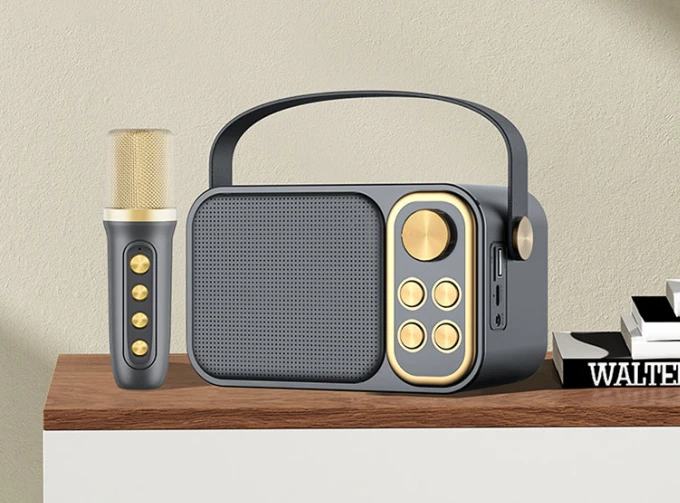Home » News » Industry News  » How much frequency response is better of the speaker?

# How much frequency response is better of the speaker?

11times   2023-02-03

Frequency response refers to the phenomenon that when an audio signal output at a constant voltage is connected to the system, the sound pressure generated by the mini bluetooth speaker increases or attenuates as the frequency changes, and the phase changes with the frequency. This sound pressure and phase The associated variation with frequency is called the frequency response. It also refers to the frequency range that the sound system can reproduce within the range allowed by the amplitude, and the amount of signal change within this range is called the frequency response, also called the frequency characteristic. Within the rated frequency range, the ratio of the maximum value to the minimum value of the output voltage amplitude is expressed in decibels (dB). In the concept of power quality, frequency response usually refers to the change of the impedance of the system or metering sensor with frequency.

How to determine the frequency response

1. Analytical method

The theoretical calculation method based on physical mechanism is only suitable for the situation where the structure and composition of the system are easy to determine. After the structural composition of the system is given, the frequency response of the system can be determined by derivation and calculation using the corresponding physical laws. The correctness of the analysis depends on the precise knowledge of the structure of the system. For complex systems, analytical methods are computationally intensive.

2. Experimental method

The method of direct measurement by instruments can be used in situations where the system structure is difficult to determine. The commonly used experimental method is to use the sinusoidal signal as the test signal, select several frequency values in the frequency range under investigation, and measure the amplitude and phase angle of the input and steady-state output sinusoidal signals at each frequency. The variation characteristic of the amplitude ratio of the output to the input with the frequency is the amplitude-frequency characteristic, and the variation characteristic of the phase angle difference between the output and the input with the frequency is the phase-frequency characteristic.Frequency Response Performance

The transition process of the system has a definite relationship with the frequency response, which can be obtained by mathematical methods. But except for first- and second-order systems, doing so often takes a lot of time, and in many cases is not practical. A common method is to directly estimate the performance of the system transition process according to the characteristic quantity of the frequency response. The main characteristic quantities of the frequency response are: gain margin and phase angle margin, resonance peak and resonance frequency, bandwidth and cut-off frequency.

Gain Margin and Phase Angle Margin

It provides information on whether the control system is stable and how much stability margin it has.

Resonant peak value Mr and resonant frequency ωr

Mr and ωr are specified as the maximum value of the amplitude-frequency characteristic |G(jω)| and the corresponding frequency value. For a high-order linear steady system with a pair of conjugate complex dominant poles (see the root locus method), when the value of Mr is in the range of (1.0-1.4) M0, a relatively satisfactory transition process performance can be obtained. where M0 is the magnitude of the frequency response when ω=0. The size of ωr represents the rapidity of the transition process: the larger the value of ωr, the better the rapidity of the output response of the system under the action of a unit step.Bandwidth and Cutoff Frequency

The cut-off frequency ωc is specified as the critical frequency when the amplitude-frequency characteristic |G(jω)| reaches 0.7M0 and continues to decline. The corresponding frequency range 0≤ω≤ωc is called the bandwidth. The meaning of the cut-off frequency is: the system has the function of filtering the signal components whose frequency is higher than ωc, while the signal components whose frequency is lower than ωc can be directly passed or slightly attenuated. From the perspective of reproducing the input signal, a larger bandwidth is often required, which corresponds to a smaller rise time and a faster response speed. But from the perspective of suppressing high-frequency noise, the bandwidth should not be too large. Therefore, determining the bandwidth requires comprehensive consideration.

First of all, understand the hearing range (frequency response) of the human ear, that is, the range of sounds that people can hear (distinguish). Normal adults are between 60Hz and 20kHz. The frequency response of audio equipment refers to the frequency range in which the equipment restores and reproduces the sound. There are differences in "grades" of audio equipment, but as a product or commodity, there are requirements for frequency response. Audio equipment that meets industrial standards should have a frequency response of 20Hz to 20kHz.

Excessively exaggerating this range has no practical significance. Normal people are already very insensitive to sounds below 60Hz or above 18kHz. The so-called "subwoofer" such as home theaters separately processes and amplifies the audio signal below 100Hz, not the real low frequency.

Frequency response is a range. The lower the lower limit, the better, preferably not higher than 20Hz; the higher the upper limit, the better, preferably not lower than 20kHz.